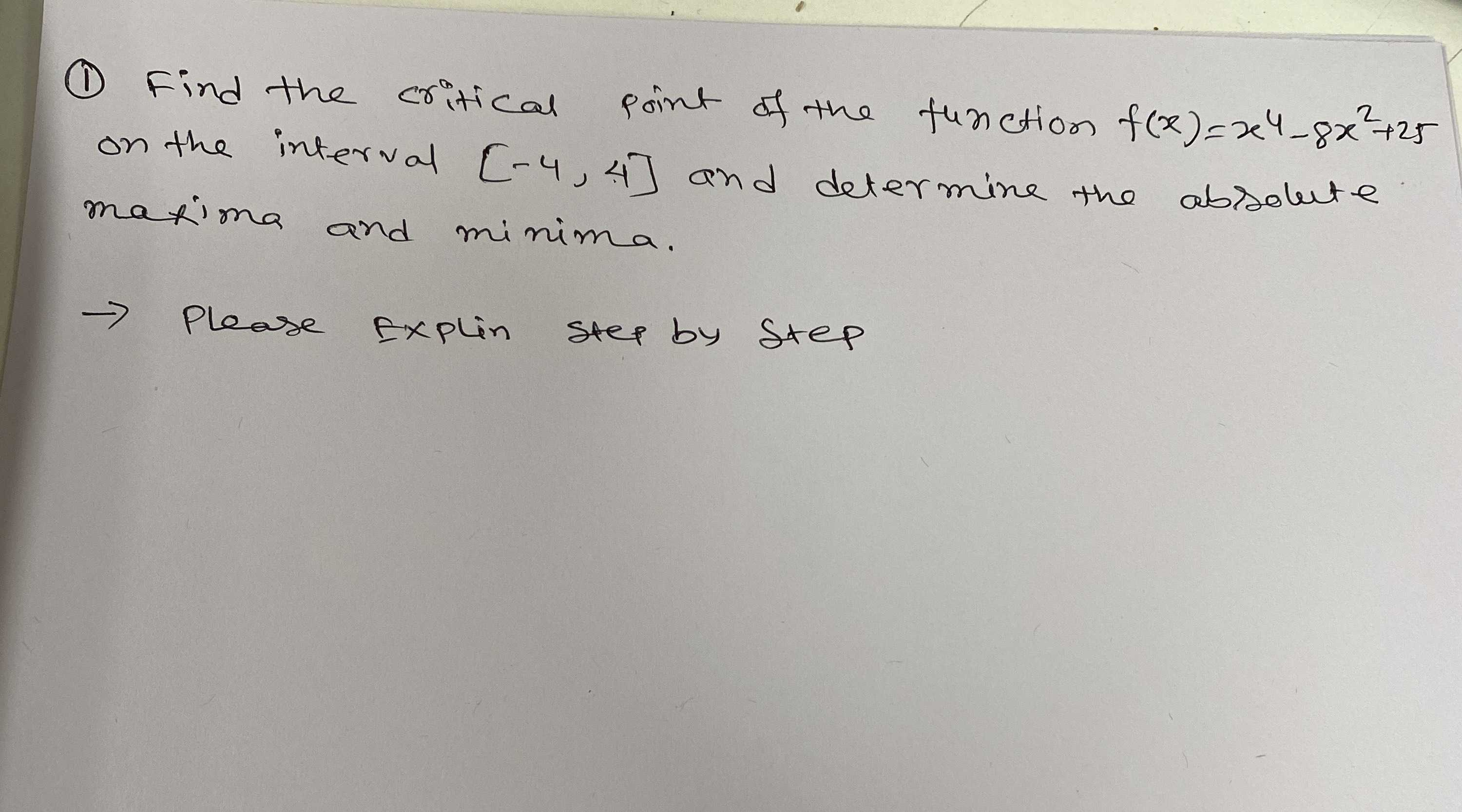### ¿Todavía tienes preguntas de matemáticas?

Pregunte a nuestros tutores expertos
Algebra
Pregunta(1) Find the critical point of the function $$f ( x ) = x ^ { 4 } - 8 x ^ { 2 } + 25$$ on the interval $$[ - 4,4 ]$$ and determine the absolute matima and minima.

$$\rightarrow$$ Please Explain step by Step